# Google Sheets Parentheses

## Parentheses

Parentheses `()` are used to change the order of an operation.

Using parentheses makes Google Sheets do the calculation for the numbers inside the parentheses first, before calculating the rest of the formula.

Parentheses are added by typing `()` on both sides of numbers, like `(1+2)`.

### Examples

No parentheses

`=10+5*2`

The result is `20` because it calculates `10+10`

With parentheses

`=(10+5)*2`

The result is `30` because it calculates `(15)*2`

Formulas can have groups of parentheses.

`=(10+5)+(2*4)+(4/2)`

Note: Cells can be used as values in the formulas inside parentheses, like `=(A1+A2)*B5`. We have used manual entries in our examples to keep things simple.

Let's have a look at some real examples in Google Sheets.

## Without Parentheses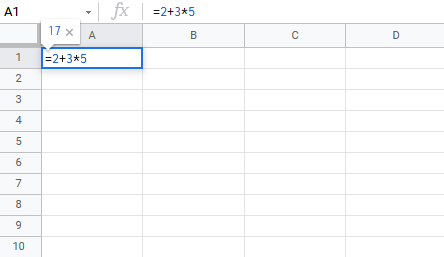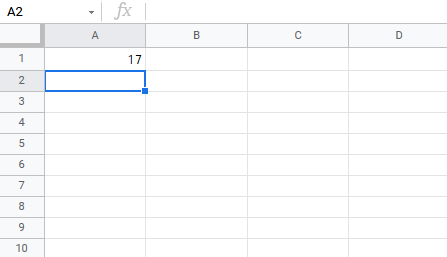The result is `17`, the calculation is `2+15`. It uses `15` because `3*5=15`.

## With One Parentheses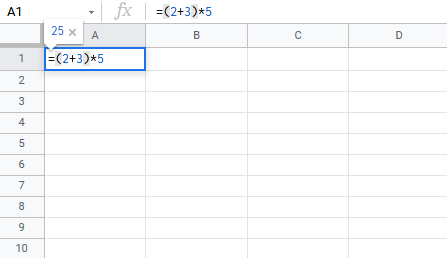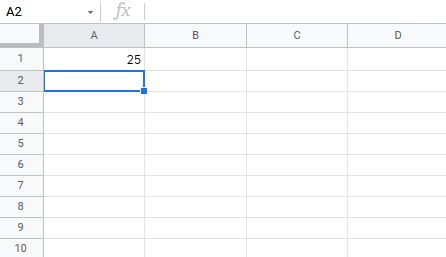The result is `25`, the calculation is `5*5`. It uses `5` because it has calculated numbers inside the parentheses `(2+3)=5` first.

## With Many Parentheses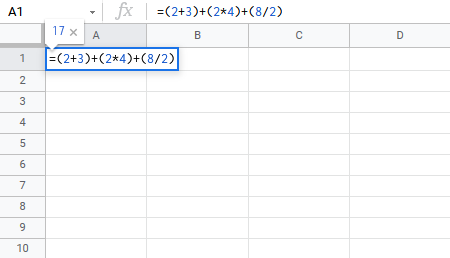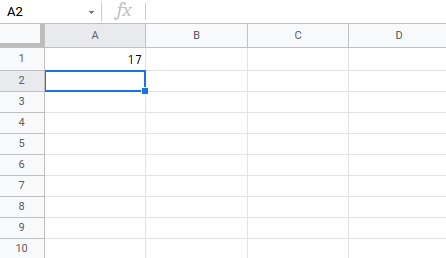The result is `17`, the calculation is `5+8+4`. The numbers inside the parentheses are calculated first.

## Nesting Parentheses

When using more advanced formulas you may need to nest parentheses. You can look at this like an onion, which has many layers. Google Sheets will calculate the numbers inside the parentheses first, layer by later, starting with the inner most layer.

Example no nesting

`=2*2+3*4+5*5*2`

It calculates the values flat as you would do with a calculator.

Example nesting

`=((2*2)+(3*4)+(5*5))*2`

Let's break it down and explain.

Nesting creates layers like an onion. You can have many layers. This example uses two, the inner and outer layers.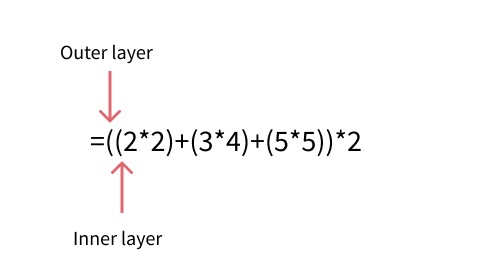It starts with calculating the numbers in the inner layer:

1. `=((2*2)+(3*4)+(5*5))*2`
2. `=(4 + 12 + 25)*2`Calculates the inner layer
3. `=(41)*2`Calculates the outer layer
4. `82`

## Chapter Summary

Parentheses can be used to change the order of an operation. The numbers inside the parentheses gets calculated first. A formula can have multiple sets of parentheses. More advanced formulas can use nesting to create layers of operations, like an onion. It calculates the inner most layer first, then the next, and so on.